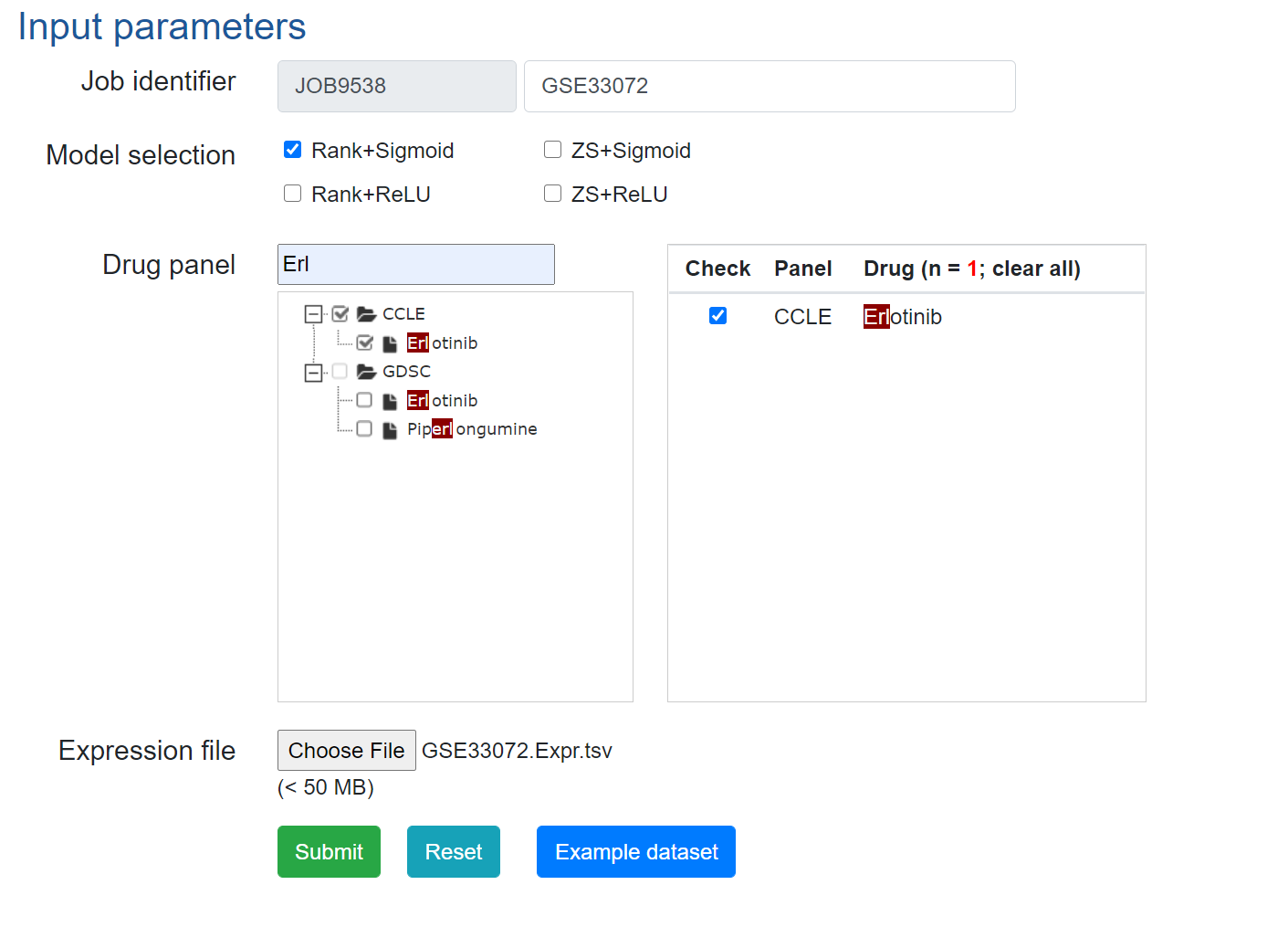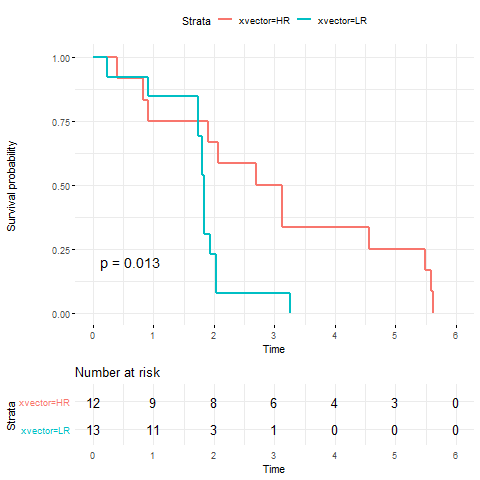## Run DrVAEN on dataset: GSE33072

#### 1. Dataset summary

Epithelial/mesenchymal transition (EMT) is associated with loss of cell adhesion molecules, such as E-cadherin, and increased invasion, migration, and proliferation in epithelial cancers. In non-small cell lung cancer (NSCLC), EMT is associated with greater resistance to EGFR inhibitors. However, its potential to predict response to other targeted drugs or chemotherapy has not been well characterized. The goal of this study was to develop a robust, platform-independent EMT gene expression signature and to investigate the association of EMT and drug response in NSCLC..

#### 2. Get and prepare dataset

The dataset can be accessed using the code:

```  #setwd("/path/to/GSE33072")
library("GEOquery")
library("limma")

gse <- getGEO("GSE33072", GSEMatrix = TRUE)

save(gse, file="GSE33072.RData")

exprs(gse[]) -> datExpr0

########## section 1
library('preprocessCore')
normalize.quantiles(datExpr0) -> datExprLQ
dimnames(datExprLQ) = dimnames(datExpr0)

########## section 2
gpl = getGEO("GPL6244")
Table(gpl) -> anno

anno\$gene_assignment -> ss
sapply(ss, function(u)trimws(strsplit(u, split="//")[])) -> x

anno = anno[which(!is.na(x)), ]
anno\$gene_assignment -> ss
sapply(ss, function(u)trimws(strsplit(u, split="//")[])) -> x
names(x) = NULL

datExprLQ = datExprLQ[which(rownames(datExprLQ) %in% anno[,1]),]

match(rownames(datExprLQ), anno[,1]) -> ii
symbol = x[ii]

apply(datExprLQ, 2, function(u)tapply(u, symbol, median)) -> expr.mat

pData(gse[]) -> pheno.anno

save(expr.mat, pheno.anno, file="GSE33072.RData")

write.table(format(expr.mat, digits=4),file="GSE33072.Expr.tsv", row.names=T, quote=F, sep="\t")
```

The genes will be mapped to our backend gene list that are used for drug response prediction. The full gene list is here

#### 3. Predict drug responses using DrVAEN.

Once the gene expression matrix file is genereted, we can upload the file to DrVAEN to get the predicted drug response data.Then download the predicted responses for further analysis.

#### 4. Further analysis: Survival analysis using the drug response data and survival data.

Here we present the example codes for the analysis.

```  library("survminer")
library("survival")

load("GSE33072.RData")
grep("progression-free survival time",pheno.anno[,"characteristics_ch1.7"]) -> ii
pheno.anno[ii, ] -> pheno.anno.pfs

########################################################################################################
ccle = read.delim("CCLE.A.pred_GSE33072.txt", as.is=T, header=T)

OS_YEAR = as.numeric(sapply(as.character(pheno.anno.pfs[,"characteristics_ch1.7"]), function(u)trimws(strsplit(u, split=":")[])))
OS = sapply( as.character(pheno.anno.pfs[,"characteristics_ch1.6"]), function(u)trimws(strsplit(u, split=":")[]))

match(pheno.anno.pfs[,2], ccle[,1]) -> ii
ccle = ccle[ii, ]

Y1 = Surv(as.numeric(OS_YEAR), as.numeric(OS))
Y_response = ccle[, "Erlotinib"]
xvector = ifelse(Y_response > median(Y_response), "HR", "LR")

fit = survfit( Surv(as.numeric(OS_YEAR), as.numeric(OS)) ~ xvector)
coxph(Y1 ~ xvector)

dat = data.frame(cbind(OS_YEAR, OS, xvector))
dat[,1] = as.numeric(as.character(dat[,1]))
fit = survfit( Surv(as.numeric(OS_YEAR), as.numeric(OS)) ~ xvector, data=dat)
g1 = ggsurvplot(fit, data=dat , risk.table = TRUE,pval = TRUE,break.time.by = 1, ggtheme = theme_minimal())

png("CCLE.png")
print(g1)
dev.off()

## To analyse the predicted drug response data from GDSC model, please just change "CCLE" to "GDSC" in the above codes.
```